Definitions of Square Dance Calls and Concepts
Good Show [C3B]

Index -->  Plus  |  A1  |  A2  |  C1  |  C2  |  C3A  |  C3B  |  C4  |  NOL  |
Definitions (Text Only) -->  Plus  |  A1  |  A2  |  C1  |  C2  |  C3A  |  C3B  |  C4  |  NOL  |
 Find call:

 \$B8@8l(B\$BE,@Z\$J(B 2 x 4 \$B\$+\$i(B.

Ends \$B\$O(B Walk & Dodge \$B\$N<+J,\$N%Q!<%H\$r9T\$\$(B, Centers \$B\$O(B Couple Up \$B\$N<+J,\$N%Q!<%H\$r9T\$\$\$^\$9(B.

2 x 4 \$B\$G=*\$o\$j\$^\$9(B.

Parallel Waves \$B\$+\$i(B: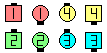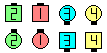Good Show\$B\$NA0(B \$B8e(B
Columns \$B\$+\$i(B: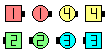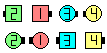Good Show\$B\$NA0(B \$B8e(B
T-Bones \$B\$+\$i(B: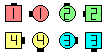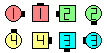Good Show\$B\$NA0(B \$B8e(B

\$BCm
• \$BA4BN\$N(B formation \$B\$r8+\$F(B End \$B\$+(B Center \$B\$+\$r7h\$a\$F(B, \$B%3!<%k\$N<+J,\$N%Q!<%H\$r(B Split \$B\$G9T\$\$\$^\$9(B.
• \$BNr;KE*\$K(B, \$B%3!<%i!<\$O(B Diagonal Box, Once Removed , Trapezoid \$B\$N\$h\$&\$J%3%s%;%W%H\$H0l=o\$K\$3\$N%3!<%k\$r;H\$\$\$^\$7\$?(B. \$BH`\$i\$O(B, \$BA4BN\$N(B 2x4 \$B\$N(B formation \$B\$G(B End \$B\$+(B Center \$B\$+\$r8+\$F(B, \$B;X<(\$5\$l\$?(B formation \$B\$G%3!<%k\$N<+J,\$N%Q!<%H\$r\$9\$k\$h\$&\$K\$7\$F\$\$\$^\$7\$?(B. \$B\$3\$l\$O(B, \$B:#\$O\$R\$s\$7\$e\$/\$rGc\$&;H\$\$J}\$G\$9(B.
• \$B1~MQ(B:Choreography for Good ShowComments? Questions? Suggestions?

https://www.ceder.net/def/goodshow.php?level=C3B&language=japan
20-January-2020 23:22:49